Pacific B usiness R eview I nternationalA Refereed Monthly International Journal of Management Indexed With THOMSON REUTERS(ESCI)
Pacific Business Review International is Included in UGC's - CARE List of Journals (Category II).
ISSN: 0974-438X
Imapct factor (SJIF): 6.56
RNI No.:RAJENG/2016/70346
Postal Reg. No.: RJ/UD/29-136/2017-2019

## Abstract

Currency trading market dominates the exchange-based trading market globally in terms of trade volume and value. The cues and shocks to the tightly integrated economies often institute short-term disequilibrium in the long-run relationship of currencies. The arbitrage trading market mechanism prevalent in an efficient market eventually corrects the disequilibrium in the currencies. We studied the co-integration and the mean-reversion in 20 international currencies, and found the cointegration in 39 currency pairs out of 139 pairs analysed. The back-testing procedure of the statistical arbitrage trading showed that the pairs trading strategy on currencies yielded positive arbitrage returns.

## 1.Introduction

### Data and Methodology

We have used the daily closing price of the spot exchange rate of 20 global currencies (quote currency) against Indian Rupee (INR is taken as base currency) over 23 years,i.e., October 1994- September 2017. The data is obtained from the Quandl database. The following exchange rates have been considered in the study viz., INR/GBP (Pound), INR/USD (US Dollar), INR/JPY (Yen), INR/CAD (Canadian Dollar), INR/CHF (Swiss Franc), INR/NZD (New Zealand Dollar), INR/SEK (Swedish Krone), INR/ NOK (Norwegian Krone), INR/BRL (Brazilian Real), INR/CNY (Chinese Yuan), INR/AUD (Australian Dollar), INR/TRY (Turkish Lira), INR/THB (Thai Baht), INR/EUR (Euro), INR/IDR (Indonesian Rupiah), NR/MYR (Malaysian Ringgit), INR/MXN (Mexican New Peso), INR/ARS (Argentinian Peso), INR/DKK (Danish Krone) and INR/ILS (Israeli Sheqel).

### Identification of currency pairs

Chen & Lin (2017) used the minimum squared distance (MSD) method to choose highly correlated equity pairs traded on the US stock markets. The MSD minimises the sum squared deviations between two normalised security prices. With the intuition that the highly correlated stocks have the same systematic risk exposure, we carried out correlation analysis. To test whether the highly correlated pairs have a co-movement, we performed a co-integration analysis on the possible combination of currencies. The number of pairs has been computed using the formula, 17C2,i.e., 136 pairs. Before the correlation analysis, we performed two analysis for removing outliers in the data viz., the graphical examination and the statistical analysis. The graphical time-series plots helped us detect a discontinuity in the time series; the box-plot helped us detect the presence of outliers. Then, we adopted the statistical technique of Inter-quartile range (IQR) to pinpoint the outliers. We computed the IQR as the difference between the upper quartile (Q3) and the lower quartile (Q1) of the currency exchange rate. The upper bound is taken as +2.5 standard deviations away from the IQR, and the lower boundis set at - 2.5 standard deviations away from the IQR, to cover about 98% of observations. The Gaussian normal distribution does not describe the observations that breach the thresholds, i.e., outliers. The IQR is representedin (1)

#### IQR = Q3 – Q1 (1)

We performed Karl Pearson correlation analysis on the currency pairs. The correlation coefficient with the cut-off of 0.7 is retained as highly correlated pairs for the study. The formula for Karl Pearson correlation is given in (2)

#### r= (n ∑▒xy- ∑▒x ∑▒y)/√((n ∑▒x^2 - 〖(∑▒〖x)〗〗^2 )(n ∑▒y^2 -(〖∑▒y)〗^2)) (2)

Where x,y represent the two-time series, and r represents the correlation coefficient. The Augmented Dickey-Fuller (ADF) test (Dickey & Fuller, 1979) is used for testing the non-stationarity of the currency time series. If yt represents a time series, when Δyt is regressed on its p-lags, if the error term μt is not white noise, then the μt is auto-correlated. The ADF tests for the unit-root of the coefficient (ψ) of the first lag of the dependent variable. The mathematical representation of the ADF test is given in (3). The null hypothesis is rejected at the 5% significance level if the ADF test statistic is less than the critical value (CV is -2.86).

### Tests for Co-integration of the currency pairs

Engle and Granger (1987) proposed that if two time-series are non-stationary and their linear combination is a stationary series, then the two time-series are co-integrated. The co-integrated series move together. The pairs trading strategy works on the idea that currency pairs are co-integrated. We used the Johansen approach (Johansen, 1988) for statistical significance of co-integrating relation between the currency pairs. The Johansen’s approach uses two test statistics namely, the trace statistic or the maximum Eigenvalue for determining the number of cointegrating relations. The trace statistic tests the joint null that the number of co-integrating vectors is less than or equal to ‘r’ (H0: r = r* < k, where r is the rank of the co-integrating matrix, k refers to the number of cointegrating relations) against an alternative that there are more than ‘r’ cointegrating vectors (Ha: r =k). The test proceeds sequentially for r* = 1,2,…k. The first non-rejection of null is the estimate of ‘r’. Similarly, the maximum Eigenvalue tests the null hypothesis that the number of co-integrating vectors is ‘r’ (H0: r =r* < k) against an alternative of ‘r + 1’ (Ha: r = r*+1) cointegration relations. The rejection of null shows the presence of a cointegrating relationship between the currencies. The mathematical representation of the trace test and the eigen value test are given in (4) and (5)

#### λ_trace= -T ∑_(i=r+1)^n▒〖ln⁡(1-(λ_i ) ̂ 〗) (4) λ_max⁡〖(r,r+1)〗 = -T ln⁡〖(1-λ ̂_(r+1) 〗) (5)

here, (λ_i ) ̂ represents the estimated eigenvalue for the co-integrating matrix.

### Estimation of the spread using an error correction model

#### Y_t- γX_t=(β_(Y_t )-γβ_(X_t ) )+ (ϵ_(Y_t )-γε_(X_t )) (6)

Where, β_(Y_t ),β_(X_t )represent the non-stationary components of Y and X respectively.〖 ϵ〗_(Y_t ), ϵ_(X_t )are errors. The residuals of the VECM should be stationary, The spread is computed as the logarithmic difference between estimated and actual exchange rates between periods t and t+i (wherei =1, 2…n) of long-short currencies. The spread is mean-reverting and stationary if the residuals of the VECM are i.i.d. The mathematical representation of the spreadis given in (7)

#### S_t= log⁡〖(1+X_t )- γ〗 log⁡〖(1+Y_(t+i))〗 (9) S_(t+i)= log⁡〖(1+Y_(t+i) )- γ〗 log⁡〖(1+X_(t+i))〗 (10) Total Ret= ∑_(i=1)^N▒(〖(S〗_t+ S_(t+i)))/2 (11)

Where N is the total number of trades The average return is computed as the total return divided by the number of trades. Trade is considered as complete if there are no openpositions,i.e., an ‘enter’ trade is squared off with a corresponding ‘exit’ trade. The average return is represented in (12)

#### Avg.Ret= (Total Ret)/N (12)

The total return and the average return are adjusted for transaction costs as per the rule of the NSE. The transaction cost at the rate of 0.042% (0.04% - exchange charges, 0.002% - clearing charges) is referred from the website Zerodha. The Hit ratio is computed as a ratio of positive trades to a total number of trades for a trading period of a currency pair. It is represented in (13)

#### Hit ratio= (No. of Positive trades)/( Total number of trades )*100 (13)

The Sharpe ratio (Sharpe, 1994) provides a measure of reward-risk for an investor. It is measured as a ratio of risk-adjusted excess return per unit of risk. It represented below.

#### Sharpe ratio= (R_p- R_f)/σ_p (14)

WhereRp denotes portfolio returns, Rf is risk-free rate and σ_p denotes the standard deviation of portfolio returns.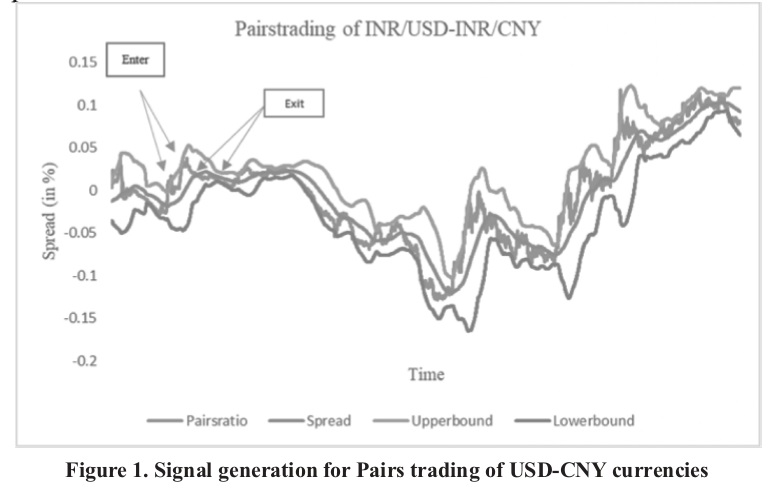### Results and Discussion

Figure. 2 show the graphical representation of global currencies. The IQR computed as in (1) showed the presence of outliers in three currencies viz., BRL, EUR, and ARS. We have removed these three currencies from further analysis as the rules of statistical arbitrage trading cannot be applied to the extreme observations.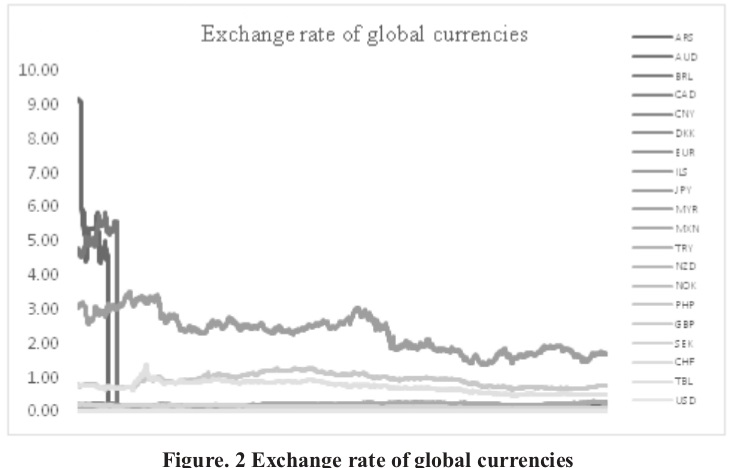Table 1 shows the correlation between currencies. The correlation coefficient (r)is computed as in (2). We have considered highly correlated currency pairs (r >=0.7 as potential pairs. We found 86 currency pairs with higher correlation. The currencies of major markets like AUD, CAD, CHF, CNY and DKK have a high correlation with all other currencies except PHP, but negative relation with TRY. The GBP has good correlation with the currencies of EU (CHF, DKK, NOK, and SEK), the US (USD, CAD), Australia(AUD), and Asia (only CNY but not with JPY). The Turkish Lira (TRY) has a negative correlation or no correlation at all with other currencies.

### Table 1: Correlation analysis of currency pairs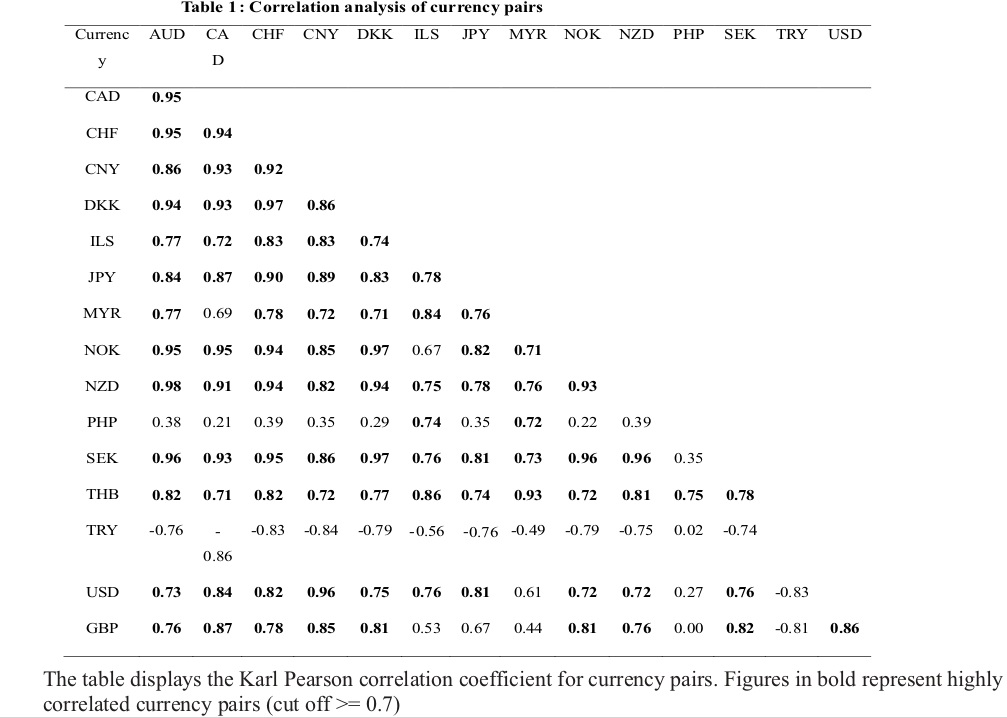Table 2 shows the results of the AugmentedDickey-Fuller test for non-stationarity of the currencies. The null hypothesis of a unit root for currencies is rejected at 5% level if the ADF statistic is less than the Dickey-Fuller critical value at 5% level of significance. It is observed that the ADF statistic for all currencies is greater than the respective critical values except for EUR. Therefore, the null is rejected only for EUR implying that EUR is stationary at level. All other currencies are non-stationary at level.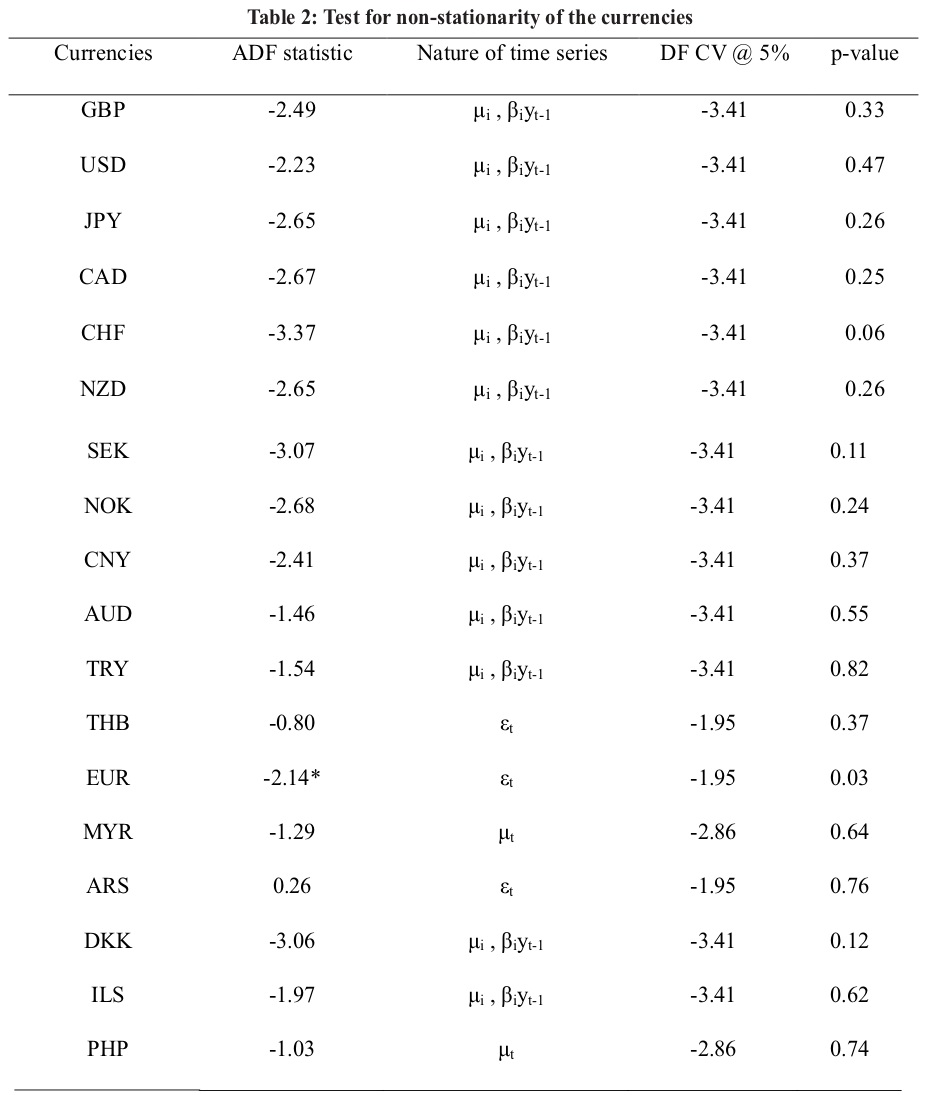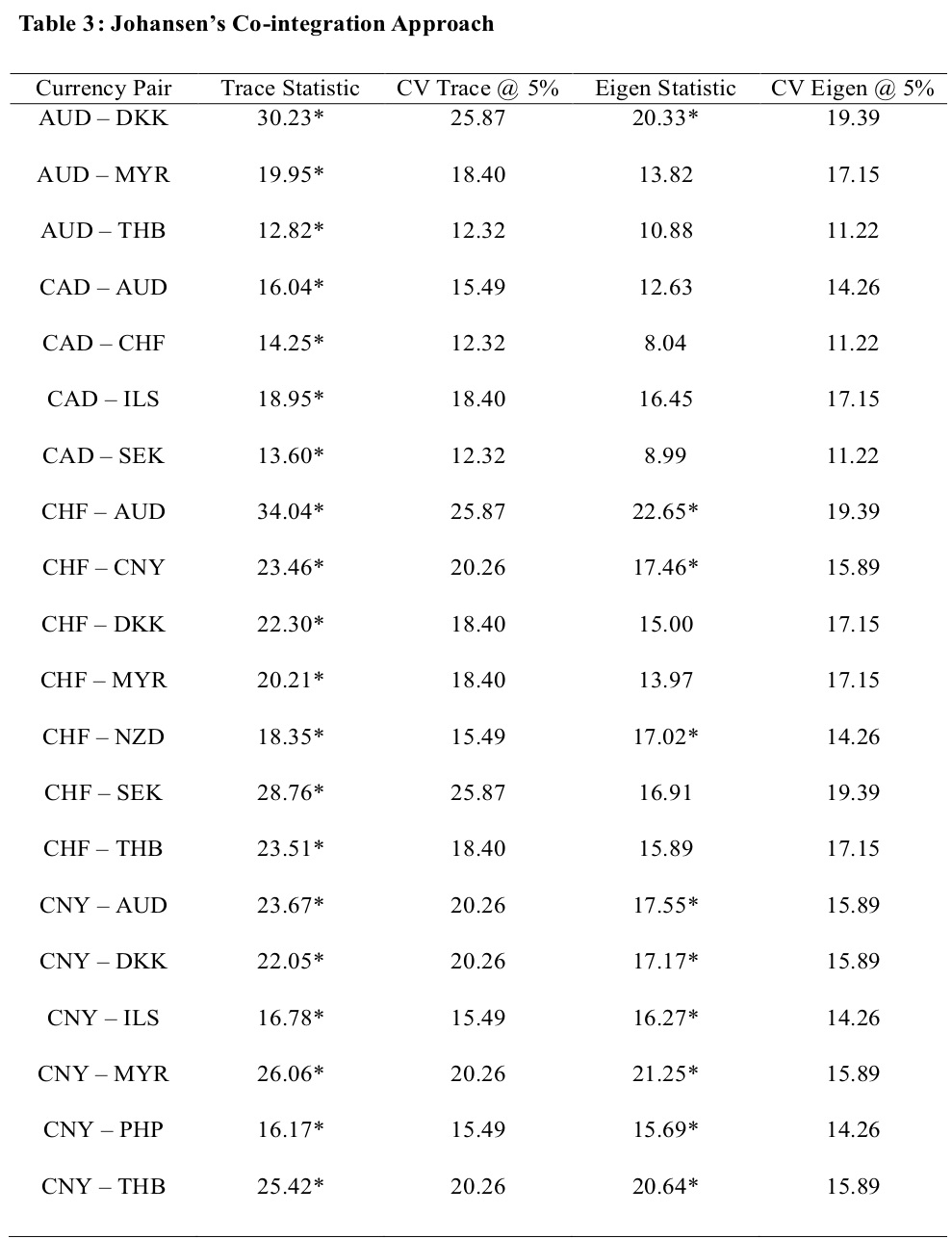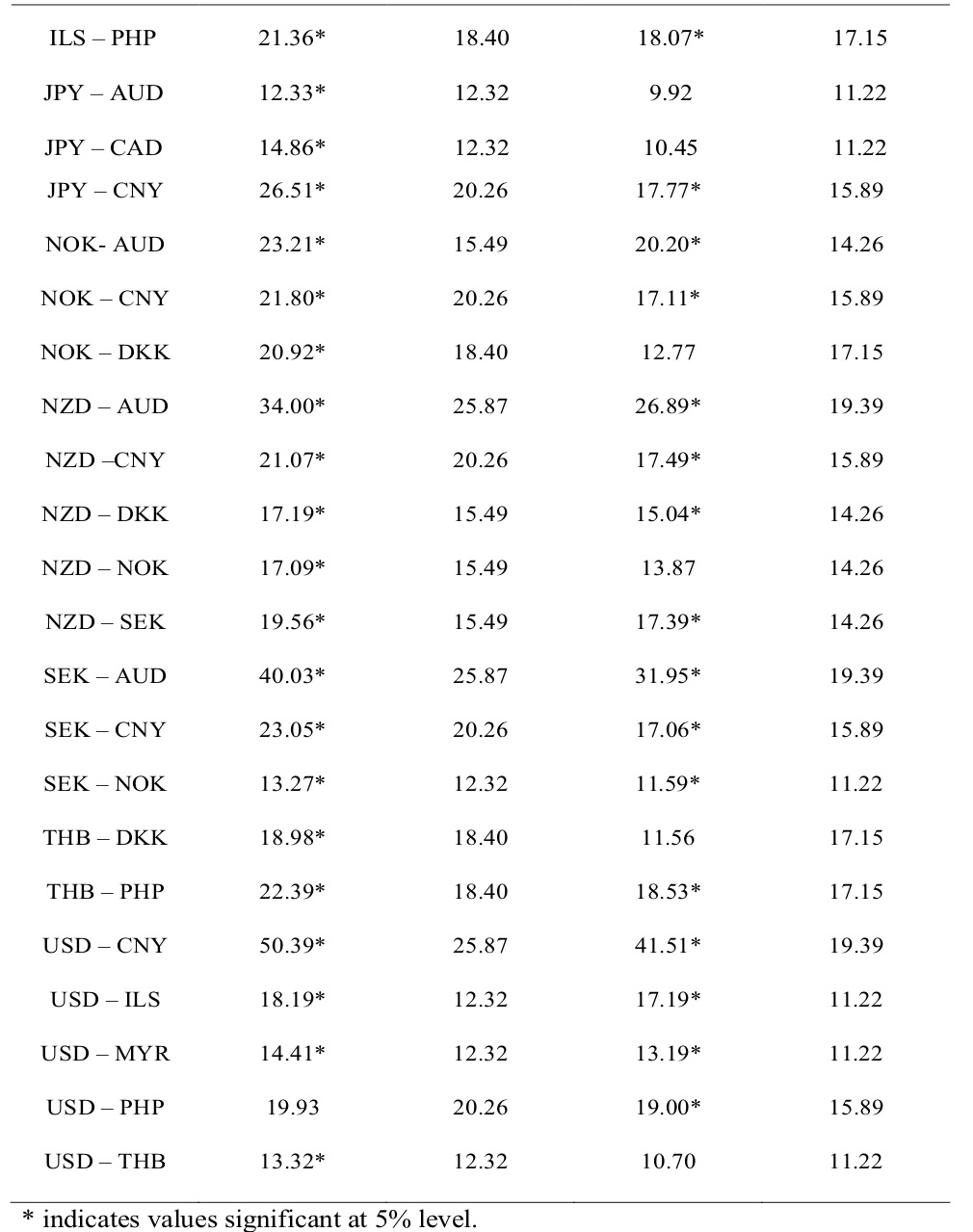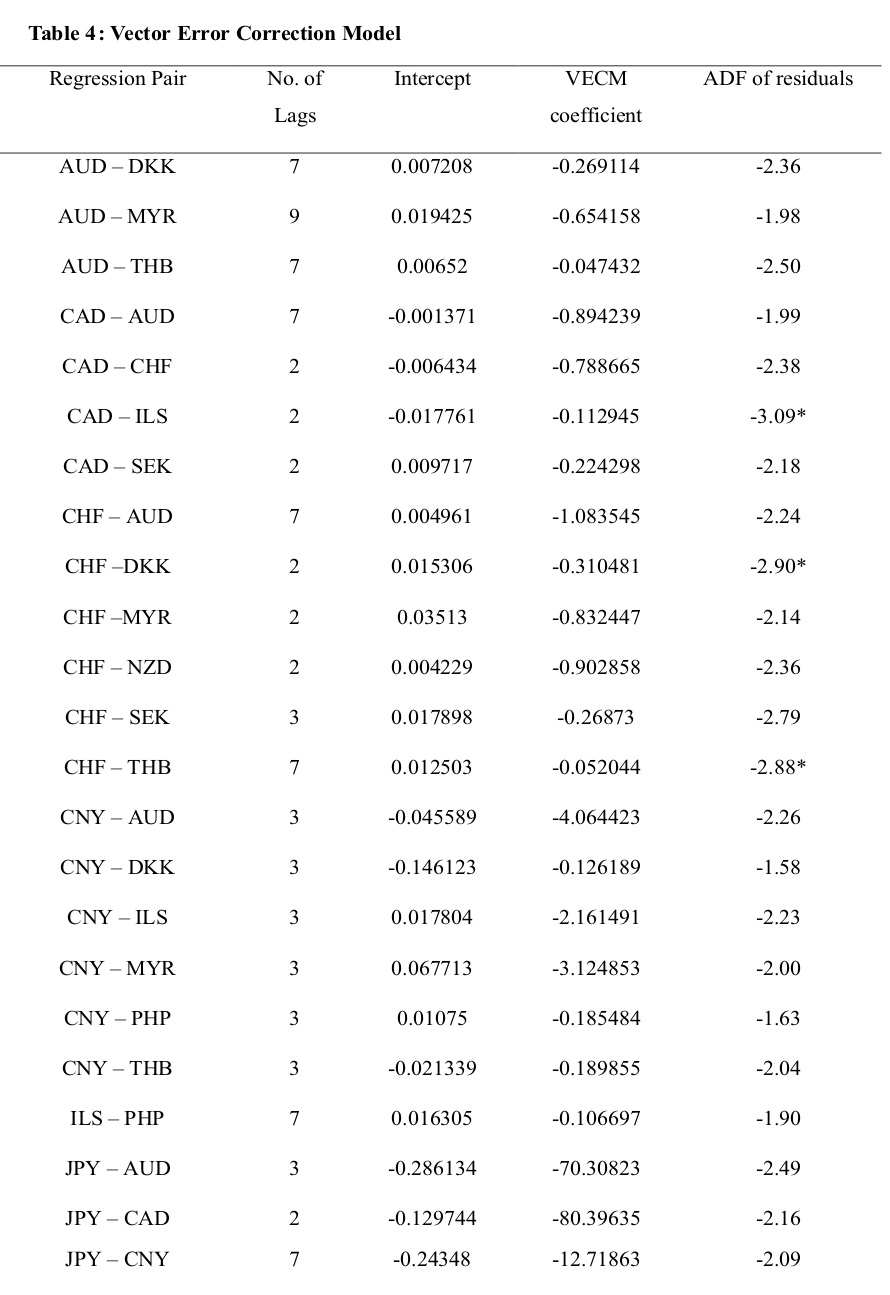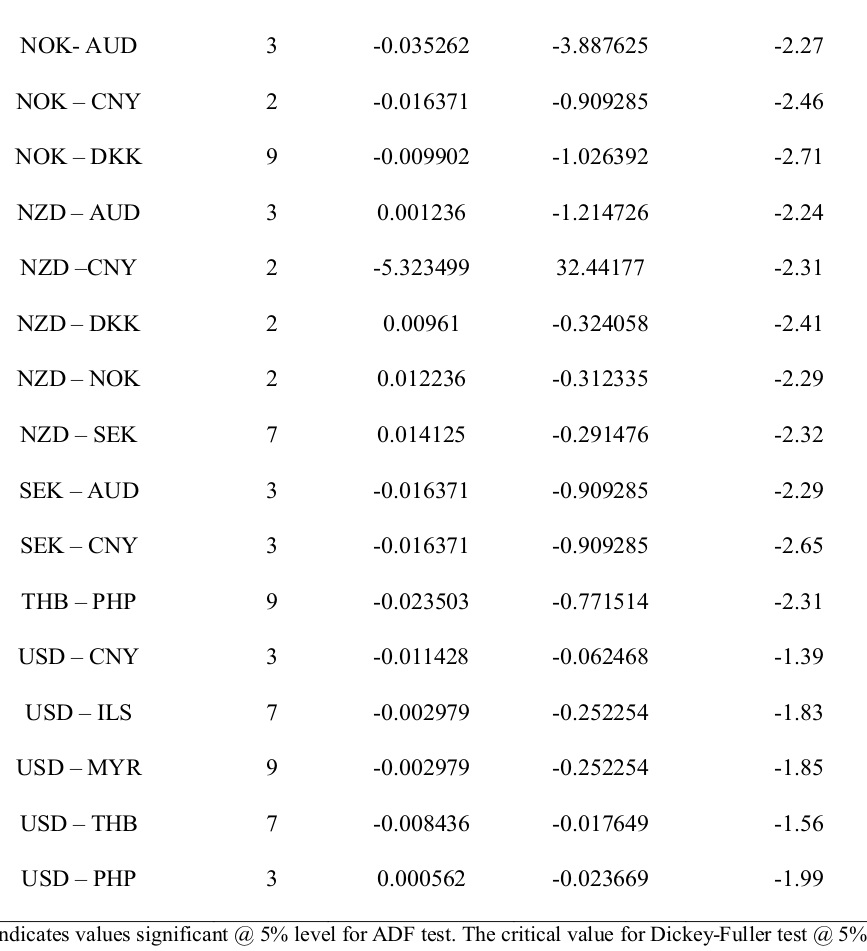is -2.86. Column 1 (Regression Pair) identify the cointegrated currency pairs. We performed the OLS regression on the pair and found that fitting an error correction model makes the regression non-spurious. Column 2 indicates the appropriate lag length used in the VECM estimation, selected based on the SIC penalty criteria. The lag with the lowest penalty criteria is the appropriate lag length. Column 3 shows the estimated intercept value from the VECM estimation. Column 4 shows the estimated VECM beta. Column 5 indicates residual diagnostics statistics. We performed the ADF test on the VECM residuals for stationarity. The VECM residuals not stationary at level followed the AR (1) order.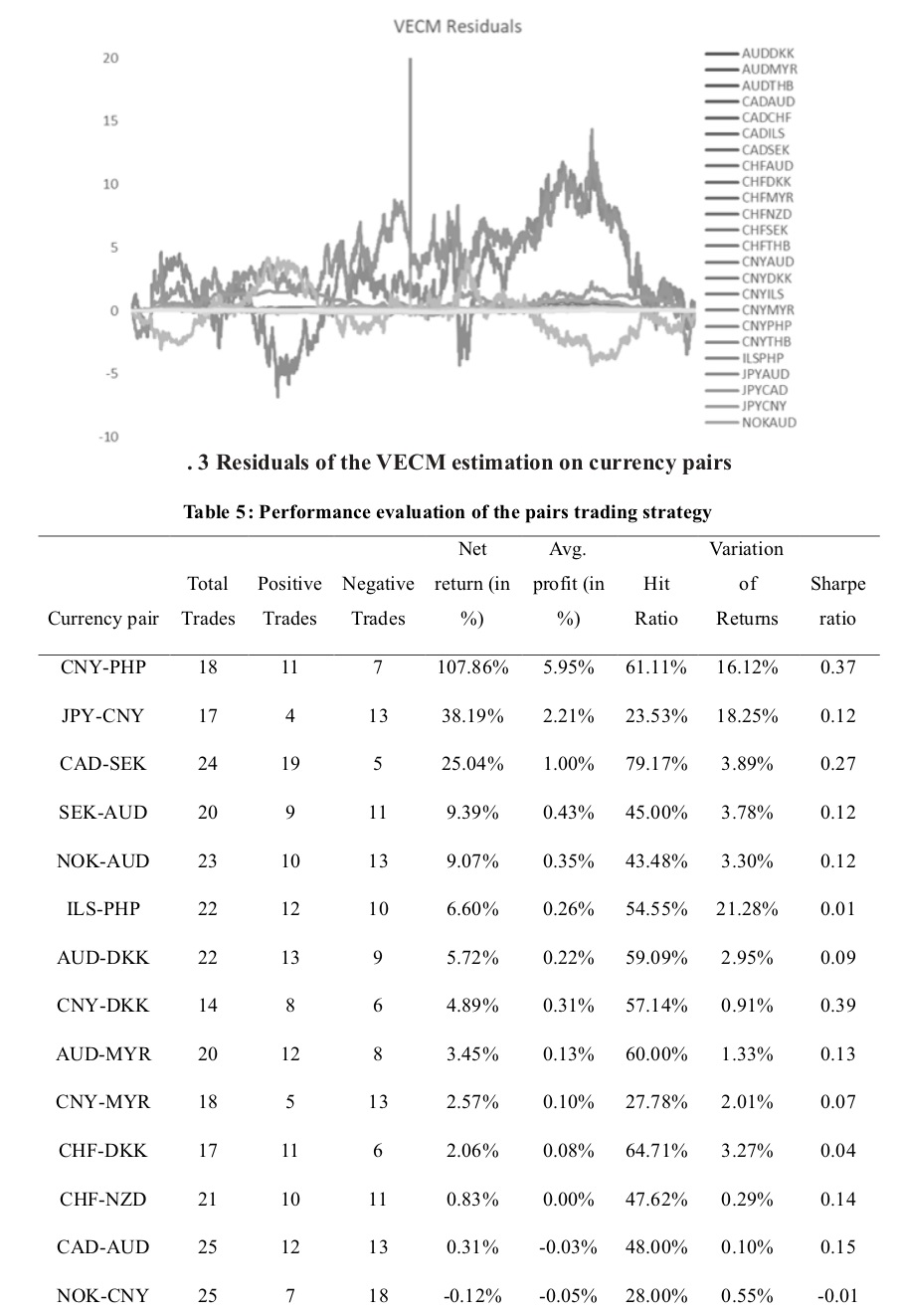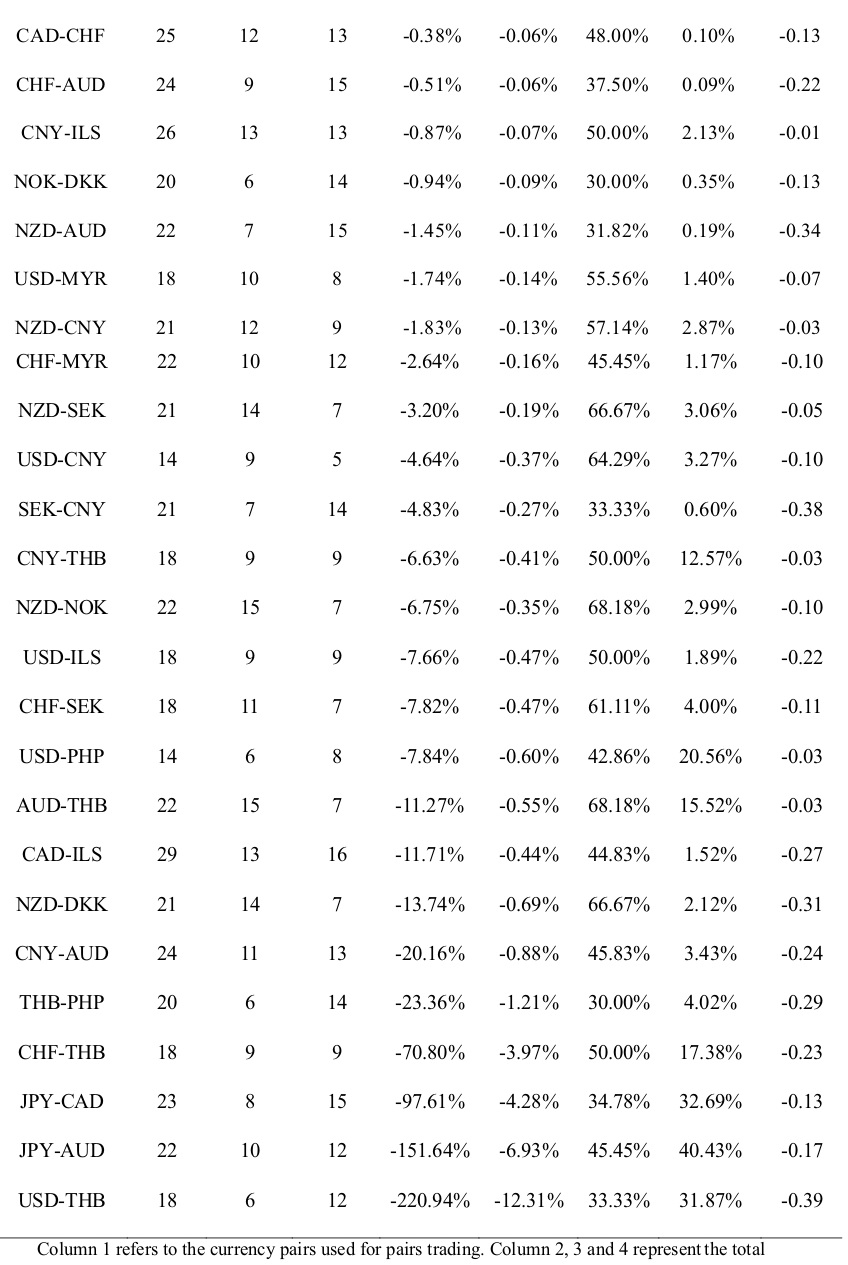Column 1 refers to the currency pairs used for pairs trading. Column 2, 3 and 4 represent the total number of trades carried out, no. of positive trades and no. of trades with negative returns, respectively, for each currency pair. Column 5 and 6 represent total return and average profit computed as in (11) and (12) respectively. The total return and the average profit are adjusted for the transaction cost available for trading on the NSE @ 00.42%. Column 7 represents the ratio of positive trades to total trades for each currency pairs,i.e. Hit ratio computed as in (13). Column 8 represents the reward-risk ratio for trading in the currency,i.e. the Sharpe ratio computed as in (14). The ‘risk-free’ rate is assumed to be 0 for calculations purpose.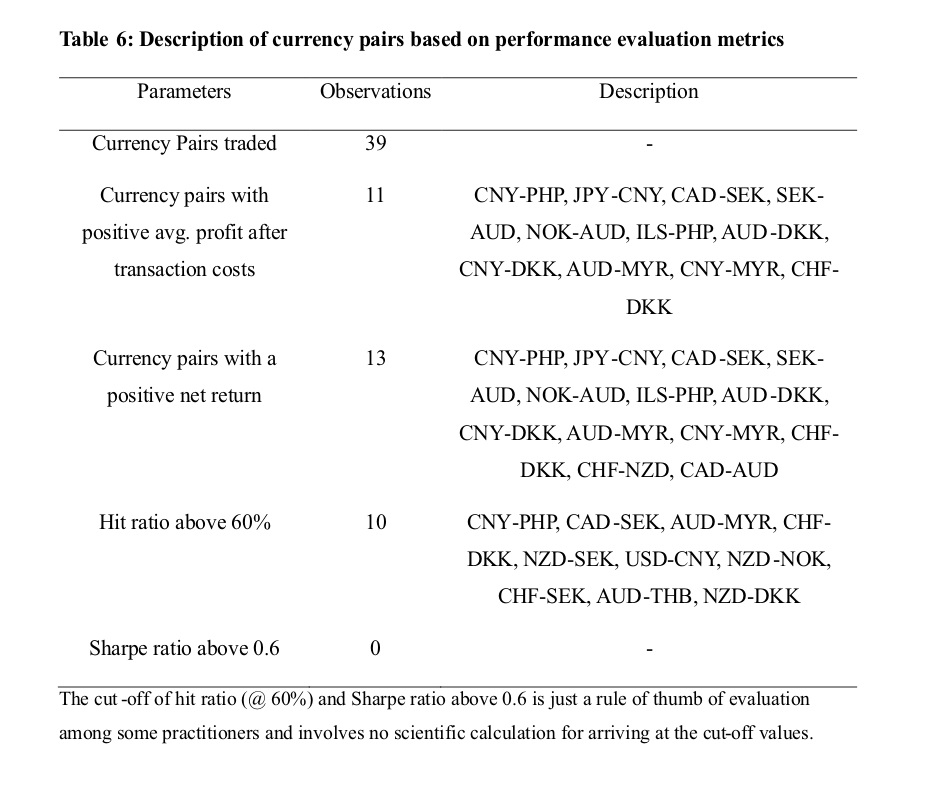### References:

```Auld, T., & Linton, O. B. (2019). The behaviour of betting and currency markets on the night of the EU referendum. International Journal Of Forecasting, 35(1), 371–389. https://doi.org/10.1016/j.ijforecast.2018.07.014
Avellaneda, M., & Lee, J. H. (2010). Statistical arbitrage in the US equities market. Quantitative Finance, 10(7), 761–782. https://doi.org/10.1080/14697680903124632
Bakhach, A. M., Tsang, E. P. K., & Raju Chinthalapati, V. L. (2018). TSFDC: A trading strategy based on forecasting directional change. Intelligent Systems in Accounting, Finance and Management, 25(3), 105–123. https://doi.org/10.1002/isaf.1425
Bakhach, A., Tsang, E., Ng, W. L., & Chinthalapati, V. L. R. (2017). Backlash Agent: A trading strategy based on Directional Change. In 2016 IEEE Symposium Series on Computational Intelligence, SSCI 2016. https://doi.org/10.1109/SSCI.2016.7850004
Chaboud, A. P., Chernenko, S. V., & Wright, J. H. (2008). Trading activity and macroeconomic announcements in high-frequency exchange rate data. Journal of the European Economic Association, 6(2–3), 589–596. https://doi.org/10.1162/JEEA.2008.6.2-3.589
Chen, C. W. S., & Lin, T. Y. (2017). Nonparametric tolerance limits for pair trading. Finance Research Letters, 21, 1–9. https://doi.org/10.1016/j.frl.2016.11.002
Choi, M. S. (2011). Momentary exchange rate locked in a triangular mechanism of international currency. Applied Economics, 43(16), 2079–2087. https://doi.org/10.1080/00036840903035969
Dickey, D. A., & Fuller, W. A. (1979). Distribution of the Estimators for Autoregressive Time Series with a Unit Root. Journal of the American Statistical Association. https://doi.org/10.2307/2286348
Elliott, R. J., der Hoek, J., & Malcolm, W. P. (2005). Pairs trading. QuantitativeFinance, 5(3), 271–276. https://doi.org/10.1201/b18325
Engle, R. F., & Granger, C. W. J. (1987). Co-Integration and Error Correction : Representation, Estimation, and Testing, Econometrica, 55(2), 251–276. https://doi.org/10.2307/1913236
Fama, E. F. (1984). Forward and spot exchange rates. Journal of Monetary Economics. https://doi.org/10.1016/0304-3932(84)90046-1
Frenkel, J. A., & Levich, R. M. (1977). Transaction Costs and Interest Arbitrage : Tranquil versus Turbulent Periods. Journal of Political Economy. https://doi.org/10.1086/260633
Galeshchuk, S. (2017). Technological bias at the exchange rate market. Intelligent Systems in Accounting, Finance and Management, 24(2–3), 80–86. https://doi.org/10.1002/isaf.1408
Galeshchuk, S., & Mukherjee, S. (2017). Deep networks for predicting the direction of change in foreign exchange rates. Intelligent Systems in Accounting, Finance and Management, 24(4), 100–110. https://doi.org/10.1002/isaf.1404
Gatev, E., Goetzmann, W. N., & Rouwenhorst, K. G. (2006). Pairs trading: Performance of a relative-value arbitrage rule. Review of Financial Studies, 19(3), 797–827. https://doi.org/10.1093/rfs/hhj020
Hansen, L. P., & Hodrick, R. J. (1980). Forward Exchange Rates as Optimal Predictors of Future Spot Rates: An Econometric Analysis. Journal of Political Economy. https://doi.org/10.1086/260910
Jacobs, H., & Weber, M. (2015). On the determinants of pairs trading profitability. Journal of Financial Markets, 23, 75–97. https://doi.org/10.1016/j.finmar.2014.12.001
Johansen, S. (1988). Statistical analysis of cointegration vectors. Journal of Economic Dynamics and Control. https://doi.org/10.1016/0165-1889(88)90041-3
Krauss, C. (2017). Statistical Arbitrage Pairs Trading Strategies: Review And Outlook.Journal of Economic Surveys. https://doi.org/10.1111/joes.12153
Neely, C. J., & Weller, P. A. (2013). Lessons from the evolution of foreign exchange trading strategies. Journal of Banking & Finance, 37(10), 3783–3798. https://doi.org/10.1016/j.jbankfin.2013.05.029
Perlin, M. S. (2009). Evaluation of pairs-trading strategy at the Brazilian financial market. Journal of Derivatives and Hedge Funds. https://doi.org/10.1057/jdhf.2009.4
Röthig, A. (2012). Cross-speculation in currency futures markets. International Journal of Finance and Economics. https://doi.org/10.1002/ijfe.462
Sharpe, W. F. (1994). The Sharpe Ratio. The Journal of Portfolio Management. https://doi.org/10.3905/jpm.1994.409501
Shleifer, A., & Vishny, R. W. (1997). The Limits of Arbitrage. The Journal of Finance. https://doi.org/10.1111/j.1540-6261.1997.tb03807.x
Vidyamurthy, G. (2004). Pairs Trading: Quantitative Methods and Analysis. John Wiley&Sons Inc.

```